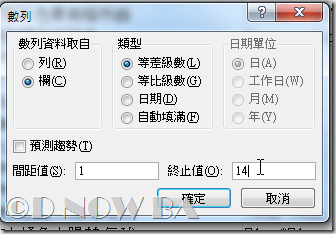## 2012年12月1日 星期六

### Excel VBA 自動流水號

Excel VBA d001d1自動流水號

##### 從錄製巨集開始
###### 操作步驟如影片###### 錄製後的程式碼如下
```Sub r001r1自動流水號()
'
' r001r1自動流水號 巨集
'

'
Selection.DataSeries Rowcol:=xlColumns, Type:=xlLinear, Date:=xlDay, _
Step:=1, Stop:=14, Trend:=False
End Sub```
• 列1：Sub 為宣告程式的開始，d001d1自動流水號()是程式的名稱。
• 列2：單引號( ' )之後的內容為註解，通常說明某一行指令的用途。
• 列7： Selection.DataSeries Rowcol:=xlColumns 中，Selection.DataSeries是使用「填滿數列」功能的指令， Rowcol是屬性，表示使用者可以填滿的方向，其值可以是xlColumns(欄)或是xlRows(列)。
• 其他的屬性及屬性值其實比對一下就知道了。這裡不贅述。
##### 開始修改程式碼
```Sub d001d1自動流水號()
'
' d001d1自動流水號 巨集
'

'
ActiveCell.Value = 1
Selection.DataSeries Rowcol:=xlColumns, Type:=xlLinear, Date:=xlDay, _
Step:=1, Stop:=InputBox("請輸入流水號的終止值"), Trend:=False
End Sub```
• 列16：ActiveCell.Value = 1表示把目前選定的儲存格填入數值1
• 列18：InputBox("請輸入流水號的終止值") 表示顯示一個對話視窗，等待使用者輸入訊息內容。
有關 InputBox的函數引數說明，請參考
InputBox Method [Excel 2007 Developer Reference]‎
##### 其他應用範例：班級座位表

Excel VBA d001d2奇偶數流水號

學校班級的座號編碼，想要以奇數或是偶數來安排，就可以設計奇、偶數數列的流水號巨集。

###### 程式碼如下
```Sub d001d2自動編號奇數()
ActiveCell.Value = 1
Selection.DataSeries Rowcol:=xlColumns, Type:=xlLinear, Date:=xlDay, _
Step:=2, Stop:=InputBox("請輸入尾數(奇數)"), Trend:=False
End Sub
Sub d001d2自動編號偶數()
ActiveCell.Value = 2
Selection.DataSeries Rowcol:=xlColumns, Type:=xlLinear, Date:=xlDay, _
Step:=2, Stop:=InputBox("請輸入尾數(偶數)"), Trend:=False
End Sub```

模組中以二個程序「自動編號奇數」和 「自動編號偶數」組合而成。注意列24、列29的地方是用step:=2，意即每次累加2的方式來自動編號

##### 其他應用範例：接續編號

Excel VBA d001d3接續編號

範例中的財產報廢表號，要讓流水號接續上一個序號，巨集是以第一個儲存格當起始值，數列接續編號到指定的數量

###### 程式碼如下
```Sub d001d3接續編號()
Selection.DataSeries Rowcol:=xlColumns, Type:=xlLinear, Date:=xlDay, _
Step:=1, Stop:=InputBox("請輸入接續編號量") + ActiveCell.Value, Trend:=False
End Sub```

列33中是和上面範例較為不同之處

#### 張貼留言

// Dnow Function